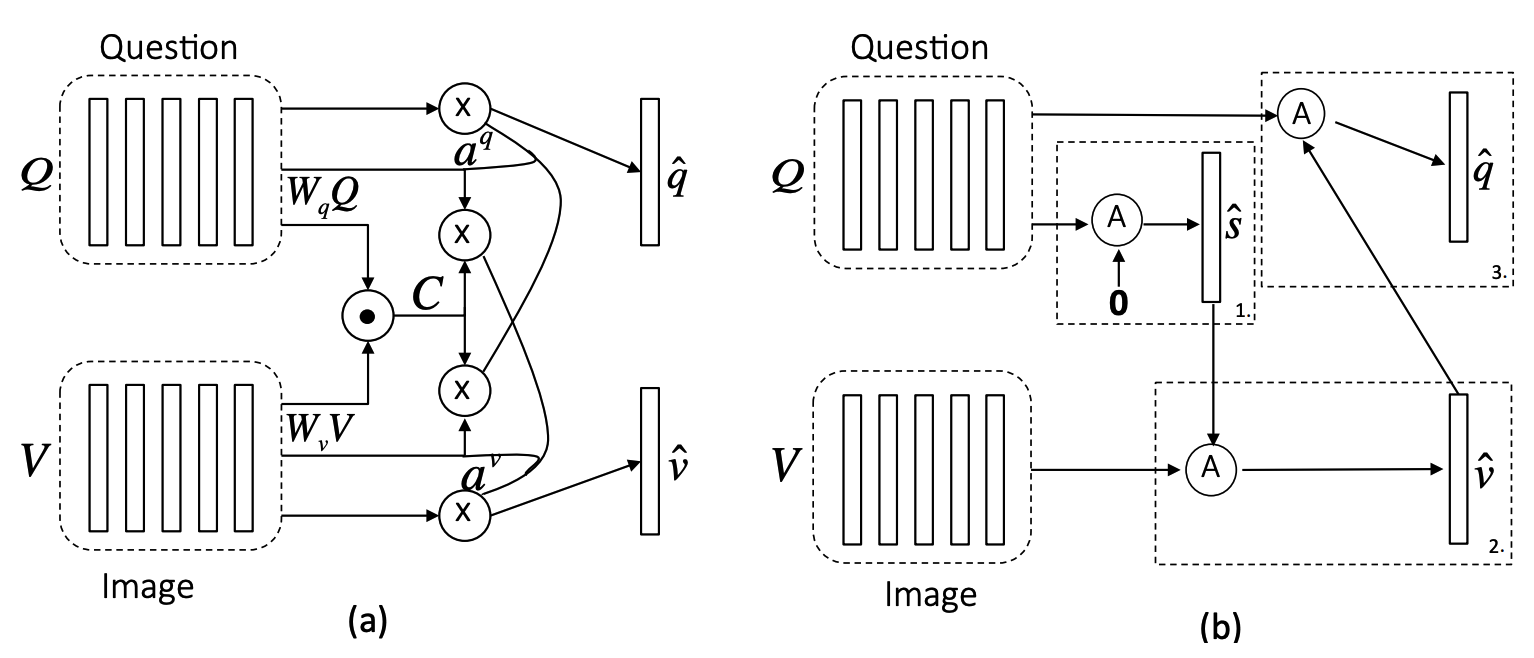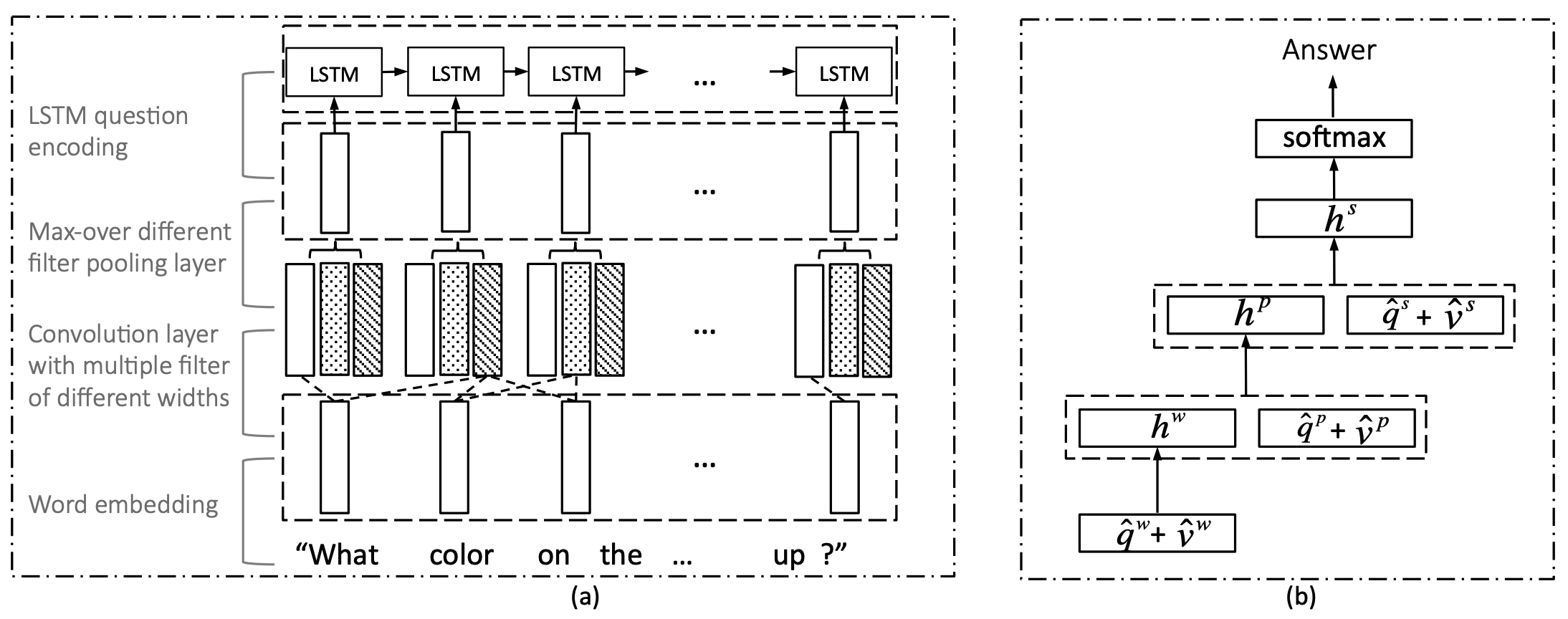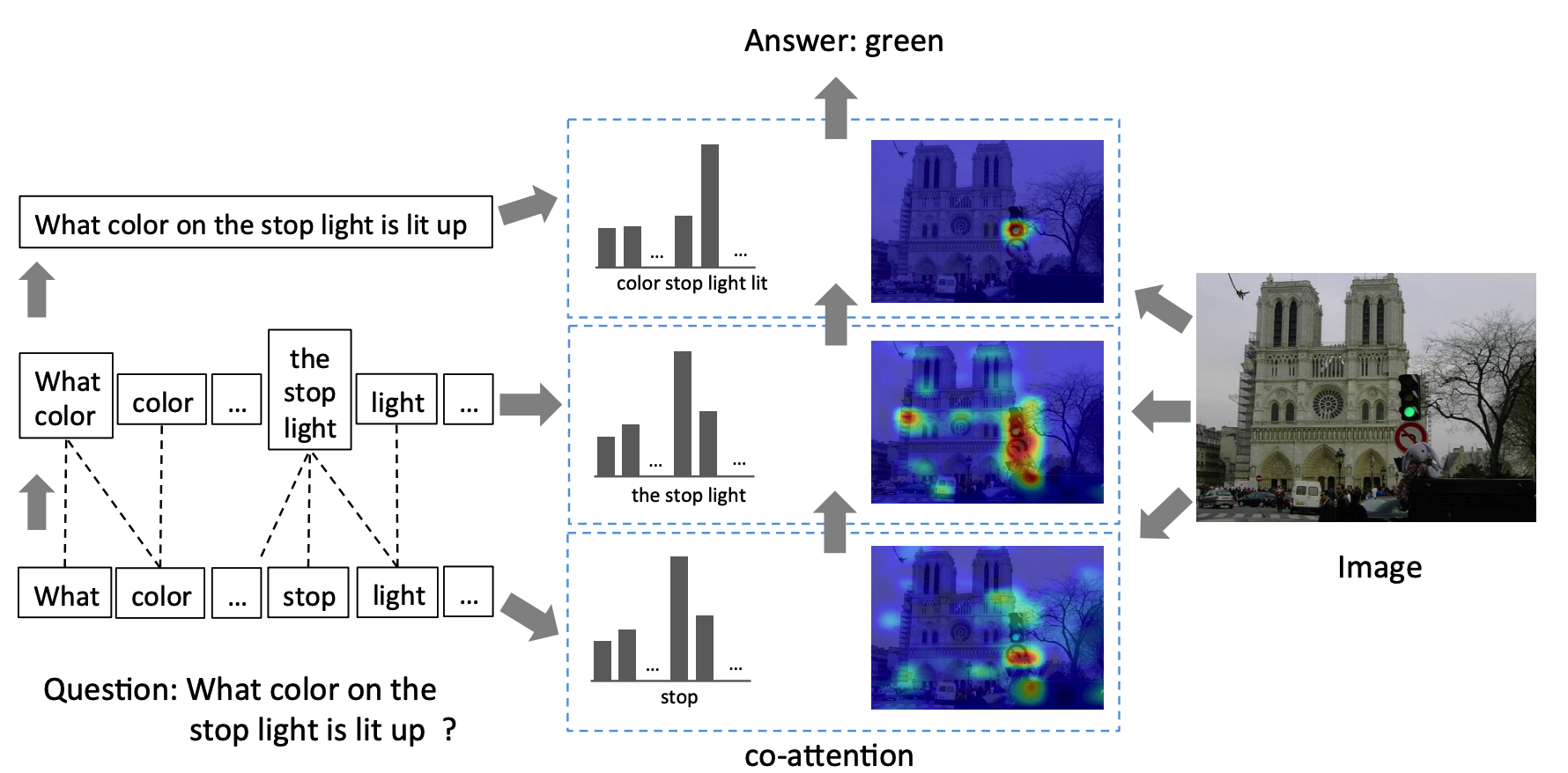# Hierarchical Question-Image Co-Attention for Visual Question Answering

## WHY?

Previous works achieved successful results in VQA by modeling visual attention. This paper suggests co-attention model for VQA to pay attention to both images (where to look) and words (what words to listen to).

## WHAT?

Co-attention model in this paper pay attention to the images and question in three level: word level, phrase level and question level. Embedding matrix is used for word level, 1D convolution with 3 window sizes(1-3) and max polling are used for phrase level, LSTM is used to encode question level vector.Two methods for co-attention are suggested: parallel co-attention and alternating co-attention. Parallell co-attention first form bilinear affinity matrix to capture the relationship between images and words and result attened visual and word features.

\mathbf{C} = tanh(\mathbf{Q}^{\top}\mathbf{W}_b\mathbf{V})\\
\mathbf{H}^v = tanh(\mathbf{W}_v\mathbf{V} + (\mathbf{W}_q\mathbf{Q})\mathbf{C}), \mathbf{H}^q = tanh(\mathbf{W}_q\mathbf{Q} + (\mathbf{W}_v\mathbf{V})\mathbf{C}^{\top})\\
\mathbf{a}^v = softmax(\mathbf{w}_{hv}^{\top}\mathbf{H}^v), \mathbf{a}^q = softmax(\mathbf{w}_{hq}^{\top}\mathbf{H}^q)\\
\hat{\mathbf{v}} = \sum_{n=1}^N a_n^v\mathbf{v}_n, \hat{\mathbf{q}} = \sum_{t=1}^T a_t^q\mathbf{q}_t

Alternating co-attention method first summerize the question into a single vector, attend to the image based on the question vector, and attend to the question based on the attened image feature. In first step, X = Q and g = 0. In second step, X = V and g = \hat{s}$g = \hat{s}$. In third step, X = Q and g = \hat{v}$g = \hat{v}$.

\hat{\mathbf{x}} = \mathcal{A}(\mathbf{X}; \mathbf{g})\\
\mathbf{H} = tanh(\mathbf{W}_x\mathbf{X} + (\mathbf{W}_g\mathbf{g)1^{\top}})\\
\mathbf{a}^x = softmax(\mathbf{w}^{\top}_{hx}\mathbf{H})\\
\hat{\mathbf{x}} = \sum a_i^x \mathbf{x}_iCo-attention is performed at various level of hierarchy of question. Various level of attended features are recursively encoded with MLP.

\mathbf{h}^w = tanh(\mathbf{W}_w(\hat{\mathbf{q}}^w + \hat{\mathbf{v}}^w))\\
\mathbf{h}^p = tanh(\mathbf{W}_p[(\hat{\mathbf{q}}^p + \hat{\mathbf{v}}^p), \mathbf{h}^w])\\
\mathbf{h}^s = tanh(\mathbf{W}_s[(\hat{\mathbf{q}}^s + \hat{\mathbf{v}}^s), \mathbf{h}^p])\\
\mathbf{p} = softmax(\mathbf{W}_h\mathbf{h}^s)

## So?Co-attention model with pretrained image feature achieved good result on VQA dataset.

Powered by aiden